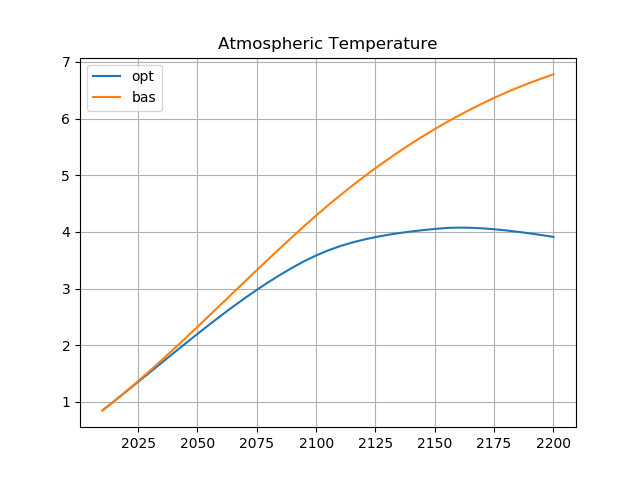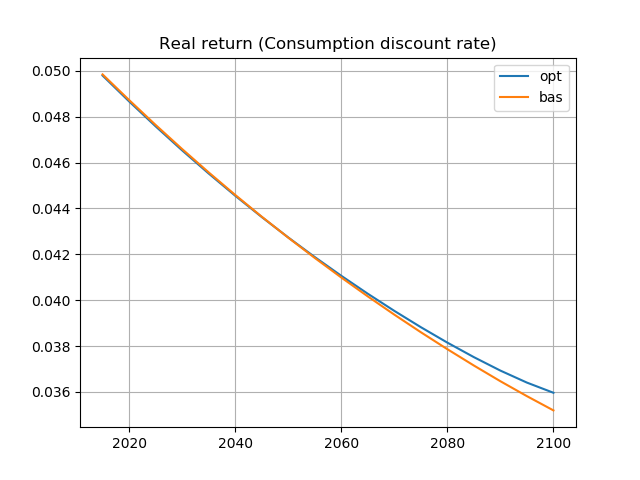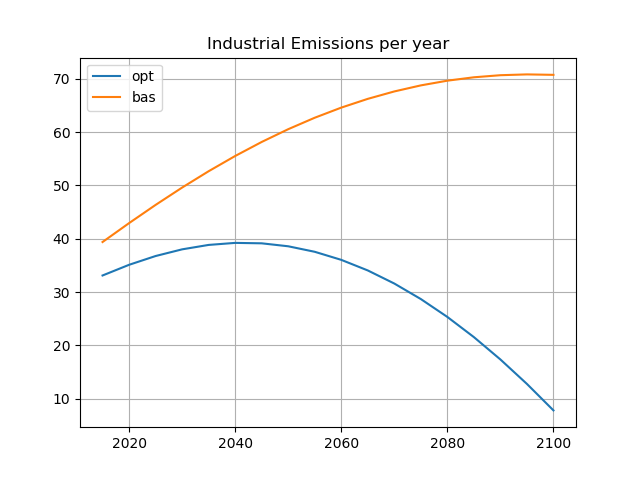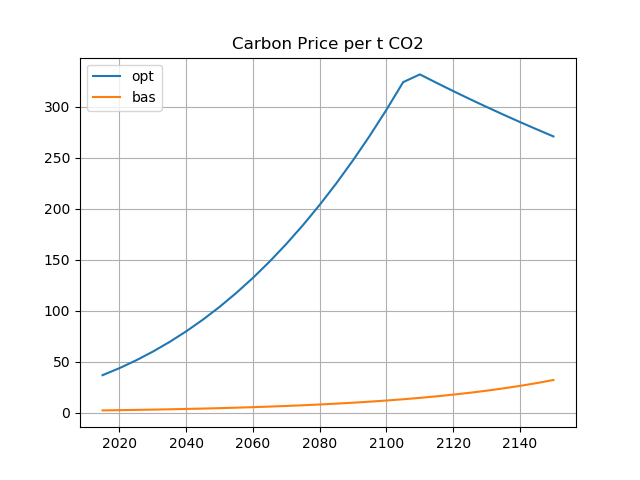William Nordhaus' DICE Model¶

The Dynamic Integrated Climate-Economy model, referred to as the DICE model or Dice model, is a computer-based integrated assessment model developed by 2018 Nobel Laureate William Nordhaus that “integrates in an end-to-end fashion the economics, carbon cycle, climate science, and impacts in a highly aggregated model that allows a weighing of the costs and benefits of taking steps to slow greenhouse warming." Nordhaus also developed the RICE model (Regional Integrated Climate-Economy model), a variant of the DICE model that was updated and developed alongside the DICE model. (source Wikipedia).

This notebook is based on the GAMS code DICE2016R-091916ap.gms provided on Nordhaus' web page.

In :
In :
%%gams

# This is the beta version of DICE-2016R. The major changes are outlined in Nordhaus,
# Revisiting the social cost of carbon: Estimates from the DICE-2016R model,"
# September 30, 2016," available from the author.

# Version is DICE-2016R-091916ap.gms

# DICE-2016R September 2016 (adapted from DICE-2016R-091216a.gms)

Set  t  'Time periods (5 years per period)'  / 1*100 / ;

Parameters
## Availability of fossil fuels
fosslim   'Maximum cumulative extraction fossil fuels (GtC)'         / 6000 /
## Time Step
tstep     'Years per Period'                                         / 5 /
## If optimal control
ifopt     'Indicator where optimized is 1 and base is 0'             / 0 /
## Preferences
elasmu    'Elasticity of marginal utility of consumption'            / 1.45 /
prstp     'Initial rate of social time preference per year'          / .015 /
## Population and technology
gama      'Capital elasticity in production function'                / .300 /
pop0      'Initial world population 2015 (millions)'                 / 7403 /
popadj    'Growth rate to calibrate to 2050 pop projection'          / 0.134 /
popasym   'Asymptotic population (millions)'                         / 11500 /
dk        'Depreciation rate on capital (per year)'                  / .100 /
q0        'Initial world gross output 2015 (trill 2010 USD)'         / 105.5 /
k0        'Initial capital value 2015 (trill 2010 USD)'              / 223 /
a0        'Initial level of total factor productivity'               / 5.115 /
ga0       'Initial growth rate for TFP per 5 years'                  / 0.076 /
dela      'Decline rate of TFP per 5 years'                          / 0.005 /
## Emissions parameters
gsigma1   'Initial growth of sigma (per year)'                       / -0.0152 /
dsig      'Decline rate of decarbonization (per period)'             / -0.001 /
eland0    'Carbon emissions from land 2015 (GtCO2 per year)'         / 2.6 /
deland    'Decline rate of land emissions (per period)'              / .115 /
e0        'Industrial emissions 2015 (GtCO2 per year)'               / 35.85 /
miu0      'Initial emissions control rate for base case 2015'        / .03 /
## Carbon cycle
# Initial Conditions
mat0      'Initial Concentration in atmosphere 2015 (GtC)'           / 851 /
mu0       'Initial Concentration in upper strata 2015 (GtC)'         / 460 /
ml0       'Initial Concentration in lower strata 2015 (GtC)'         / 1740 /
mateq     'Equilibrium concentration atmosphere (GtC)'               / 588 /
mueq      'Equilibrium concentration in upper strata (GtC)'          / 360 /
mleq      'Equilibrium concentration in lower strata (GtC)'          / 1720 /
# Flow paramaters
b12       'Carbon cycle transition matrix'                           / .12 /
b23       'Carbon cycle transition matrix'                           / 0.007 /
# These are for declaration and are defined later
b11       'Carbon cycle transition matrix'
b21       'Carbon cycle transition matrix'
b22       'Carbon cycle transition matrix'
b32       'Carbon cycle transition matrix'
b33       'Carbon cycle transition matrix'
sig0      'Carbon intensity 2010 (kgCO2 per output 2005 USD 2010)'
## Climate model parameters
t2xco2    'Equilibrium temp impact (oC per doubling CO2)'            / 3.1 /
fex0      '2015 forcings of non-CO2 GHG (Wm-2)'                      / 0.5 /
fex1      '2100 forcings of non-CO2 GHG (Wm-2)'                      / 1.0 /
tocean0   'Initial lower stratum temp change (C from 1900)'          / .0068 /
tatm0     'Initial atmospheric temp change (C from 1900)'            / 0.85 /
c1        'Climate equation coefficient for upper level'             / 0.1005 /
c3        'Transfer coefficient upper to lower stratum'              / 0.088 /
c4        'Transfer coefficient for lower level'                     / 0.025 /
fco22x    'Forcings of equilibrium CO2 doubling (Wm-2)'              / 3.6813 /
## Climate damage parameters
a10       'Initial damage intercept'                                 / 0 /
a1        'Damage intercept'                                         / 0 /
a2        'Damage quadratic term'                                    / 0.00236 /
a3        'Damage exponent'                                          / 2.00 /
## Abatement cost
expcost2  'Exponent of control cost function'                        / 2.6 /
pback     'Cost of backstop 2010$per tCO2 2015' / 550 / gback 'Initial cost decline backstop cost per period' / .025 / limmiu 'Upper limit on control rate after 2150' / 1.2 / tnopol 'Period before which no emissions controls base' / 45 / cprice0 'Initial base carbon price (2010$ per tCO2)'               / 2 /
gcprice   'Growth rate of base carbon price per year'                / .02 /

## Scaling and inessential parameters
# Note that these are unnecessary for the calculations
# They ensure that MU of first period's consumption =1 and PV cons = PV utilty
scale1    'Multiplicative scaling coefficient'               / 0.0302455265681763 /
scale2    'Additive scaling coefficient'                     / -10993.704 /
;

# Program control variables
Sets  tfirst(t), tlast(t), tearly(t), tlate(t) ;

Parameters
l(t)          'Level of population and labor'
al(t)         'Level of total factor productivity'
sigma(t)      'CO2-equivalent-emissions output ratio'
rr(t)         'Average utility social discount rate'
ga(t)         'Growth rate of productivity from'
forcoth(t)    'Exogenous forcing for other greenhouse gases'
gl(t)         'Growth rate of labor'
gcost1        'Growth of cost factor'
gsig(t)       'Change in sigma (cumulative improvement of energy efficiency)'
etree(t)      'Emissions from deforestation'
cumetree(t)   'Cumulative from land'
lam           'Climate model parameter'
gfacpop(t)    'Growth factor population'
pbacktime(t)  'Backstop price'
optlrsav      'Optimal long-run savings rate used for transversality'
scc(t)        'Social cost of carbon'
cpricebase(t) 'Carbon price in base case'
photel(t)     'Carbon Price under no damages (Hotelling rent condition)'
ppm(t)        'Atmospheric concentrations parts per million'
atfrac(t)     'Atmospheric share since 1850'
atfrac2010(t) 'Atmospheric share since 2010'
;

# Program control definitions
tfirst(t) = yes$(t.val eq 1); tlast(t) = yes$(t.val eq card(t));
# Parameters for long-run consistency of carbon cycle
b11 = 1 - b12;
b21 = b12*MATEQ/MUEQ;
b22 = 1 - b21 - b23;
b32 = b23*mueq/mleq;
b33 = 1 - b32;
# Further definitions of parameters
a20 = a2;
sig0 = e0/(q0*(1-miu0));
lam = fco22x/ t2xco2;
l("1") = pop0;
loop(t, l(t+1)=l(t););

ga(t) = ga0*exp(-dela*5*((t.val-1)));
al("1") = a0;          loop(t, al(t+1)=al(t)/((1-ga(t))););
gsig("1") = gsigma1;   loop(t,gsig(t+1)=gsig(t)*((1+dsig)**tstep) ;);
sigma("1") = sig0;     loop(t,sigma(t+1)=(sigma(t)*exp(gsig(t)*tstep)););

pbacktime(t) = pback*(1-gback)**(t.val-1);
cost1(t) = pbacktime(t)*sigma(t)/expcost2/1000;

etree(t) = eland0*(1-deland)**(t.val-1);
cumetree("1") = 100;    loop(t,cumetree(t+1)=cumetree(t)+etree(t)*(5/3.666););

rr(t) = 1/((1+prstp)**(tstep*(t.val-1)));
forcoth(t) = fex0+ (1/17)*(fex1-fex0)*(t.val-1)$(t.val lt 18) + (fex1-fex0)$(t.val ge 18);
optlrsav = (dk + .004)/(dk + .004*elasmu + prstp)*gama;

# Base Case Carbon Price
cpricebase(t) = cprice0*(1+gcprice)**(5*(t.val-1));

Variables
MIU(t)           'Emission control rate GHGs'
FORC(t)          'Increase in radiative forcing (watts per m2 from 1900)'
TATM(t)          'Increase temperature of atmosphere (degrees C from 1900)'
TOCEAN(t)        'Increase temperatureof lower oceans (degrees C from 1900)'
MAT(t)           'Carbon concentration increase in atmosphere (GtC from 1750)'
MU(t)            'Carbon concentration increase in shallow oceans (GtC from 1750)'
ML(t)            'Carbon concentration increase in lower oceans (GtC from 1750)'
E(t)             'Total CO2 emissions (GtCO2 per year)'
EIND(t)          'Industrial emissions (GtCO2 per year)'
C(t)             'Consumption (trillions 2005 US dollars per year)'
K(t)             'Capital stock (trillions 2005 US dollars)'
CPC(t)           'Per capital consumption (thousands 2005 USD per year)'
I(t)             'Investment (trillions 2005 USD per year)'
S(t)             'Gross savings rate as fraction of gross world product'
RI(t)            'Real interest rate (per annum)'
Y(t)             'Gross world product net of abatement and damages (trillions 2005 USD per year)'
YGROSS(t)        'Gross world product GROSS of abatement and damages (trillions 2005 USD per year)'
YNET(t)          'Output net of damages equation (trillions 2005 USD per year)'
DAMAGES(t)       'Damages (trillions 2005 USD per year)'
DAMFRAC(t)       'Damages as fraction of gross output'
ABATECOST(t)     'Cost of emissions reductions  (trillions 2005 USD per year)'
MCABATE(t)       'Marginal cost of abatement (2005$per ton CO2)' CCA(t) 'Cumulative industrial carbon emissions (GTC)' CCATOT(t) 'Total carbon emissions (GtC)' PERIODU(t) 'One period utility function' CPRICE(t) 'Carbon price (2005$ per ton of CO2)'
CEMUTOTPER(t)    'Period utility'
UTILITY          'Welfare function'
;

NonNegative Variables  MIU, TATM, MAT, MU, ML, Y, YGROSS, C, K, I ;

Equations
# Emissions and Damages
EEQ(t)           'Emissions equation'
EINDEQ(t)        'Industrial emissions'
CCACCA(t)        'Cumulative industrial carbon emissions'
CCATOTEQ(t)      'Cumulative total carbon emissions'
DAMFRACEQ(t)     'Equation for damage fraction'
DAMEQ(t)         'Damage equation'
ABATEEQ(t)       'Cost of emissions reductions equation'
MCABATEEQ(t)     'Equation for MC abatement'
CARBPRICEEQ(t)   'Carbon price equation from abatement'

# Climate and carbon cycle
MMAT(t)          'Atmospheric concentration equation'
MMU(t)           'Shallow ocean concentration'
MML(t)           'Lower ocean concentration'
TATMEQ(t)        'Temperature-climate equation for atmosphere'
TOCEANEQ(t)      'Temperature-climate equation for lower oceans'

# Economic variables
YGROSSEQ(t)      'Output gross equation'
YNETEQ(t)        'Output net of damages equation'
YY(t)            'Output net equation'
CC(t)            'Consumption equation'
CPCE(t)          'Per capita consumption definition'
SEQ(t)           'Savings rate equation'
KK(t)            'Capital balance equation'
RIEQ(t)          'Interest rate equation'

# Utility
CEMUTOTPEREQ(t)  'Period utility'
PERIODUEQ(t)     'Instantaneous utility function equation'
UTIL             'Objective function'
;

## Equations of the model
# Emissions and Damages
eeq(t)..             E(t)          =E= EIND(t) + etree(t);
eindeq(t)..          EIND(t)       =E= sigma(t) * YGROSS(t) * (1-(MIU(t)));
ccacca(t+1)..        CCA(t+1)      =E= CCA(t) + EIND(t)*5/3.666;
ccatoteq(t)..        CCATOT(t)     =E= CCA(t) + cumetree(t);
force(t)..           FORC(t)       =E= fco22x * ((log((MAT(t)/588.000))/log(2))) + forcoth(t);
damfraceq(t) ..      DAMFRAC(t)    =E= (a1*TATM(t)) + (a2*TATM(t)**a3) ;
dameq(t)..           DAMAGES(t)    =E= YGROSS(t) * DAMFRAC(t);
abateeq(t)..         ABATECOST(t)  =E= YGROSS(t) * cost1(t) * (MIU(t)**expcost2);
mcabateeq(t)..       MCABATE(t)    =E= pbacktime(t) * MIU(t)**(expcost2-1);
carbpriceeq(t)..     CPRICE(t)     =E= pbacktime(t) * (MIU(t))**(expcost2-1);

# Climate and carbon cycle
mmat(t+1)..          MAT(t+1)      =E= MAT(t)*b11 + MU(t)*b21 + (E(t)*(5/3.666));
mml(t+1)..           ML(t+1)       =E= ML(t) *b33  + MU(t)*b23;
mmu(t+1)..           MU(t+1)       =E= MAT(t)*b12 + MU(t)*b22 + ML(t)*b32;
tatmeq(t+1)..        TATM(t+1)     =E= TATM(t) + c1*((FORC(t+1)-(fco22x/t2xco2)*TATM(t))-(c3*(TATM(t)-TOCEAN(t))));
toceaneq(t+1)..      TOCEAN(t+1)   =E= TOCEAN(t) + c4*(TATM(t)-TOCEAN(t));

# Economic variables
ygrosseq(t)..        YGROSS(t)     =E= (al(t)*(L(t)/1000)**(1-GAMA))*(K(t)**GAMA);
yneteq(t)..          YNET(t)       =E= YGROSS(t)*(1-damfrac(t));
yy(t)..              Y(t)          =E= YNET(t) - ABATECOST(t);
cc(t)..              C(t)          =E= Y(t) - I(t);
cpce(t)..            CPC(t)        =E= 1000 * C(t) / L(t);
seq(t)..             I(t)          =E= S(t) * Y(t);
kk(t+1)..            K(t+1)        =L= (1-dk)**tstep * K(t) + tstep * I(t);
rieq(t+1)..          RI(t)         =E= (1+prstp) * (CPC(t+1)/CPC(t))**(elasmu/tstep) - 1;

# Utility
cemutotpereq(t)..    CEMUTOTPER(t) =E= PERIODU(t) * L(t) * rr(t);
periodueq(t)..       PERIODU(t)    =E= ((C(T)*1000/L(T))**(1-elasmu)-1)/(1-elasmu)-1;
util..               UTILITY       =E= tstep * scale1 * sum(t, CEMUTOTPER(t)) + scale2;

# Resource limit
CCA.up(t) = fosslim;

# Control rate limits
MIU.up(t)            = limmiu;
MIU.up(t)$(t.val<30) = 1; ## Upper and lower bounds for stability K.LO(t) = 1; MAT.LO(t) = 10; MU.LO(t) = 100; ML.LO(t) = 1000; C.LO(t) = 2; TOCEAN.UP(t) = 20; TOCEAN.LO(t) = -1; TATM.UP(t) = 20; CPC.LO(t) = .01; TATM.UP(t) = 12; # Control variables set lag10(t); lag10(t) = yes$(t.val gt card(t)-10);
S.FX(lag10(t)) = optlrsav;

# Initial conditions
CCA.FX(tfirst)    = 400;
K.FX(tfirst)      = k0;
MAT.FX(tfirst)    = mat0;
MU.FX(tfirst)     = mu0;
ML.FX(tfirst)     = ml0;
TATM.FX(tfirst)   = tatm0;
TOCEAN.FX(tfirst) = tocean0;

## Solution options
model CO2 / all /;

Optimized Run¶

In :
%%gams
MIU.FX('1') = miu0;
solve CO2 maximizing UTILITY using nlp;
Out:
Solver Status Model Status Objective #equ #var Model Type Solver Solver Time
0 Normal (1) OptimalLocal (2) 4517.3147 2493 2700 NLP CONOPT 0.851
In :
%gams_pull -d TATM RI EIND CPRICE
%gams_pull tstep t
In :
import pandas as pd

def fillDF(orgDF,newDF,col):
for j in zip(orgDF.level.index,orgDF.level):
newDF.loc[2010+tstep*j,col] = j

df = {}
for d in ['TATM','RI','EIND','CPRICE']:
df[d] = pd.DataFrame(columns=['opt','bas'])
fillDF(eval(d),df[d],'opt')

Base Run¶

In :
%%gams
# For base run, this subroutine calculates Hotelling rents
# Carbon price is maximum of Hotelling rent or baseline price
# The cprice equation is different from 2013R. Not sure what went wrong.
option bRatio = 1; MIU.LO('1') = 0; MIU.UP('1') = inf; # Reset fixing from opt run and discard basis
a2 = 0;
solve CO2 maximizing UTILITY using nlp;
photel(t) = CPRICE.L(t);
a2 = a20;
CPRICE.UP(t)\$(t.val<tnopol+1) = max(photel(t),cpricebase(t));
option bRatio=0.25;
solve CO2 maximizing UTILITY using nlp;
Out:
Solver Status Model Status Objective #equ #var Model Type Solver Solver Time
0 Normal (1) OptimalLocal (2) 4620.3546 2493 2700 NLP CONOPT 0.292
1 Normal (1) OptimalLocal (2) 4485.7435 2493 2700 NLP CONOPT 0.372
In :
%gams_pull -d TATM RI EIND CPRICE
In :
for d in ['TATM','RI','EIND','CPRICE']:
fillDF(eval(d),df[d],'bas')

Visualization¶

In :
%matplotlib notebook
plot = df['TATM'].loc[2000:2200,:].plot(grid=True,title="Atmospheric Temperature")In :
plot = df['RI'].loc[2015:2100,:].plot(grid=True,title="Real return (Consumption discount rate)")In :
plot = df['EIND'].loc[2015:2100,:].plot(grid=True,title="Industrial Emissions per year")In :
plot = df['CPRICE'].loc[2015:2150,:].plot(grid=True,title="Carbon Price per t CO2")Post Processing¶

In :
%%gams
## POST-SOLVE
# Calculate social cost of carbon and other variables
scc(t) = -1000*eeq.m(t)/(.00001+cc.m(t));
atfrac(t) = ((MAT.L(t)-588)/(CCATOT.L(t)+.000001  ));
atfrac2010(t) = ((MAT.L(t)-mat0)/(.00001+CCATOT.L(t)-CCATOT.L('1')  ));
ppm(t) = MAT.L(t)/2.13;

Parameter rep(*,t);
rep("Industrial Emissions GTCO2 per year", t) = EIND.L(t);
rep("Atmospheric concentration C (ppm)", t) = MAT.L(t)/2.13;
rep("Atmospheric Temperature", t) = TATM.L(t);
rep("Output Net Net)", t) = Y.L(t);
rep("Climate Damages fraction output", t) = DAMFRAC.L(t);
rep("Consumption Per Capita", t) = CPC.L(t);
rep("Carbon Price (per t CO2)", t) = CPRICE.L(t);
rep("Emissions Control Rate", t) = MIU.L(t);
rep("Social cost of carbon", t) = scc(t);
rep("Interest Rate", t) = RI.L(t);
rep("Population", t) = l(t);
rep("TFP", t) = al(t);
rep("Output gross,gross", t) = YGROSS.L(t);
rep("Change tfp", t) = ga(t);
rep("Capital", t) = K.L(t);
rep("s", t) = S.L(t);
rep("I", t) = I.L(t);
rep("Y gross net", t) = YNET.L(t);
rep("damages", t) = DAMAGES.L(t);
rep("damfrac", t) = DAMFRAC.L(t);
rep("abatement", t) = ABATECOST.L(t);
rep("sigma", t) = sigma(t);
rep("Forcings", t) = FORC.L(t);
rep("Other Forcings", t) = forcoth(t);
rep("Period utilty", t) = PERIODU.L(t);
rep("Consumption", t) = C.L(t);
rep("Land emissions", t) = etree(t);
rep("Cumulative ind emissions", t) = CCA.L(t);
rep("Cumulative total emissions", t) = CCATOT.L(t);
rep("Atmospheric concentrations Gt", t) = MAT.L(t);
rep("Atmospheric concentrations ppm", t) = ppm(t);
rep("Total Emissions GTCO2 per year", t) = E.L(t);
rep("Atmospheric concentrations upper", t) = MU.L(t);
rep("Atmospheric concentrations lower", t) = ML.L(t);
rep("Atmospheric fraction since 1850", t) = atfrac(t);
rep("Atmospheric fraction since 2010", t) = atfrac2010(t);
In :
%gams_pull -d rep
def gt_pivot(df, index=None, columns=None, values=None):
df = df.pivot(index=index, columns=columns, values=values)
df.index.names = [None]
df.columns.names = [None]
return df

# Uncomment the next two lines to see the entire table
#pd.set_option('display.max_columns', None)  # or 1000
#pd.set_option('display.max_rows', None)  # or 1000

# Rename periods to years
rep['t'] = rep['t'].map({s:2010+(int(tstep)*int(s)) for s in t})
gt_pivot(rep,'t','*','value')
Out:
Atmospheric Temperature Atmospheric concentration C (ppm) Atmospheric concentrations Gt Atmospheric concentrations lower Atmospheric concentrations ppm Atmospheric concentrations upper Atmospheric fraction since 1850 Atmospheric fraction since 2010 Capital Carbon Price (per t CO2) ... Population Social cost of carbon TFP Total Emissions GTCO2 per year Y gross net abatement damages damfrac s sigma
2015 0.850000 399.530516 851.000000 1740.000000 399.530516 460.000000 0.526000 NaN 223.000000 2.000000 ... 7403.000000 3.123105e+01 5.115000 38.344714 104.998084 0.000847 0.179338 0.001705 0.259555 0.350320
2020 1.016345 418.468429 891.337755 1740.670698 418.468429 471.289302 0.549229 0.771309 267.942171 2.208162 ... 7853.090848 3.725411e+01 5.535714 41.684564 125.041570 0.001116 0.305569 0.002438 0.256250 0.324682
2025 1.189237 438.311198 933.602851 1741.419438 438.311198 485.128389 0.567352 0.756778 318.424941 2.437989 ... 8264.920660 4.403940e+01 5.978891 44.967870 147.277995 0.001456 0.493217 0.003338 0.253388 0.301035
2030 1.367930 459.148661 977.986648 1742.263955 459.148661 501.231051 0.581651 0.744870 374.617056 2.691737 ... 8638.974960 5.162013e+01 6.444808 48.151900 171.746414 0.001881 0.761815 0.004416 0.250919 0.279215
2035 1.551747 481.006178 1024.543158 1743.219953 481.006178 519.392164 0.593004 0.734869 436.677524 2.971895 ... 8976.556918 6.002761e+01 6.933693 51.200920 198.479057 0.002410 1.134340 0.005683 0.248797 0.259074
2040 1.740021 503.864308 1073.230976 1744.301678 503.864308 539.454754 0.602033 0.726275 504.755542 3.281212 ... 9279.542985 6.929121e+01 7.445717 54.085599 227.501967 0.003065 1.637270 0.007145 0.246979 0.240476
2045 1.932077 527.672719 1123.942892 1745.522256 527.672719 561.288761 0.609196 0.718736 578.990163 3.622723 ... 9550.179528 7.943816e+01 7.981001 56.782340 258.835493 0.003870 2.300530 0.008810 0.245427 0.223296
2050 2.127227 552.360041 1176.526887 1746.893885 552.360041 584.777682 0.614843 0.712003 659.509819 3.999779 ... 9790.919966 9.049342e+01 8.539609 59.272601 292.494667 0.004857 3.157335 0.010679 0.244110 0.207420
2055 2.324774 577.840873 1230.801059 1748.427926 577.840873 609.810442 0.619245 0.705898 746.431809 4.416079 ... 10004.298976 1.024796e+02 9.121553 61.542225 328.489512 0.006061 4.243947 0.012755 0.242996 0.192744
2060 2.524023 604.020777 1286.564255 1750.134949 604.020777 636.276699 0.622620 0.700291 839.861822 4.875708 ... 10192.838895 1.154166e+02 9.726788 63.580827 366.825298 0.007525 5.599341 0.015035 0.242062 0.179171
2065 2.724292 630.799846 1343.603671 1752.024734 630.799846 664.064391 0.625141 0.695088 939.893530 5.383176 ... 10358.982970 1.293218e+02 10.355217 65.381227 407.502767 0.009300 7.264808 0.017515 0.241285 0.166615
2070 2.924924 658.075250 1401.700283 1754.106265 658.075250 693.058680 0.626953 0.690217 1046.608262 5.943461 ... 10505.050289 1.442098e+02 11.006691 66.938947 450.518339 0.011445 9.283503 0.020190 0.240646 0.154996
2075 3.125292 685.743067 1460.632732 1756.387706 685.743067 723.141772 0.628172 0.685626 1160.074704 6.562062 ... 10633.207677 1.600925e+02 11.681006 68.251763 495.864303 0.014029 11.699957 0.023051 0.240127 0.144238
2080 3.324808 713.699579 1520.180102 1758.876386 713.699579 754.193232 0.628895 0.681273 1280.348535 7.245046 ... 10745.454538 1.769792e+02 12.377909 69.319317 543.528984 0.017136 14.559559 0.026088 0.239715 0.134275
2085 3.522925 741.842204 1580.123895 1761.578781 741.842204 786.090577 0.629204 0.677125 1407.471779 7.999116 ... 10843.617248 1.948772e+02 13.097098 70.142775 593.496866 0.020862 17.908029 0.029290 0.239394 0.125046
2090 3.719144 770.070147 1640.249413 1764.500497 770.070147 818.709975 0.629163 0.673157 1541.471481 8.831671 ... 10929.350409 2.137922e+02 13.838222 70.724528 645.748632 0.025321 21.790890 0.032644 0.239153 0.116492
2095 3.913009 798.284842 1700.346714 1767.646268 798.284842 851.926978 0.628828 0.669346 1682.356928 9.750878 ... 11004.142809 2.337296e+02 14.600884 71.067920 700.261018 0.030646 26.252925 0.036135 0.238978 0.108561
2100 4.104112 826.390244 1760.211219 1771.019950 826.390244 885.617214 0.628246 0.665675 1830.113960 10.765758 ... 11069.326443 2.546969e+02 15.384645 71.176997 757.006323 0.036993 31.337637 0.039751 0.238855 0.101206
2105 4.289134 854.292976 1819.644039 1774.624520 854.292976 919.657016 0.627453 0.662128 1984.693527 11.886266 ... 11126.087363 2.767158e+02 16.189020 71.056250 816.002302 0.044546 37.035609 0.043416 0.238795 0.094382
2110 4.468130 881.902364 1878.452036 1778.462088 881.902364 953.923958 0.626482 0.658691 2146.175925 13.123398 ... 11175.477434 2.998057e+02 17.013490 70.712159 877.242225 0.053518 43.375342 0.047115 0.238791 0.088050
2115 4.641151 909.131500 1936.450094 1782.533902 909.131500 988.297293 0.625359 0.655354 2314.617687 14.489292 ... 11218.426348 3.239841e+02 17.857493 70.151100 940.715532 0.064161 50.382537 0.050835 0.238834 0.082170
2120 4.808240 935.896873 1993.460339 1786.840364 935.896873 1022.658573 0.624106 0.652104 2490.058303 15.997349 ... 11255.753449 3.492675e+02 18.720437 69.379393 1006.408812 0.076768 58.079817 0.054561 0.238921 0.076710
2125 4.969437 962.118144 2049.311647 1791.381045 962.118144 1056.892052 0.622740 0.648934 2672.524850 17.662366 ... 11288.179053 3.756717e+02 19.601694 68.403304 1074.306519 0.091682 66.486469 0.058281 0.239046 0.071637
2130 5.124784 987.717975 2103.839287 1796.154707 987.717975 1090.884945 0.621276 0.645834 2862.035279 19.500680 ... 11316.335091 4.032114e+02 20.500608 67.229026 1144.391510 0.109300 75.618223 0.061982 0.239206 0.066922
2135 5.274321 1012.621896 2156.884638 1801.159327 1012.621896 1124.527591 0.619728 0.642795 3058.600821 21.530326 ... 11340.774957 4.319013e+02 21.416497 65.862647 1216.645473 0.130089 85.487041 0.065652 0.239398 0.062539
2140 5.418088 1036.758180 2208.294922 1806.392112 1036.758180 1157.713554 0.618103 0.639811 3262.227837 23.771220 ... 11361.982524 4.617554e+02 22.348652 64.310114 1291.049296 0.154592 96.100939 0.069279 0.239618 0.058462
2145 5.556127 1060.057721 2257.922946 1811.849532 1060.057721 1190.339668 0.616410 0.636873 3472.919318 26.245347 ... 11380.380319 4.927875e+02 23.296344 62.577195 1367.583394 0.183439 107.463829 0.072855 0.239864 0.054670
2150 5.688481 1082.453908 2305.626823 1817.527340 1082.453908 1222.306039 0.614654 0.633973 3690.676182 28.976984 ... 11396.336877 5.250113e+02 24.258825 60.669445 1446.228017 0.217365 119.575384 0.076367 0.240135 0.051141
2155 5.815190 1103.882486 2351.269694 1823.420593 1103.882486 1253.516021 0.612841 0.631106 3915.498438 31.992932 ... 11410.173332 5.584399e+02 25.235328 58.592174 1526.963556 0.257226 132.430921 0.079807 0.240429 0.047856
2160 5.936296 1124.281418 2394.719421 1829.523682 1124.281418 1283.876155 0.610973 0.628264 4147.386311 35.322782 ... 11422.169274 5.930865e+02 26.225075 56.350417 1609.770841 0.304015 146.021300 0.083165 0.240744 0.044796
... ... ... ... ... ... ... ... ... ... ... ... ... ... ... ... ... ... ... ... ... ...
2365 7.010705 1107.313183 2358.577081 2140.869290 1107.313183 1438.259455 0.522802 0.522248 18646.189219 125.134131 ... 11499.785783 2.491161e+03 69.059363 -5.677858 6446.702704 1.639746 845.897699 0.115994 0.249687 0.003893
2370 6.998055 1103.146827 2349.702742 2147.800484 1103.146827 1432.458658 0.521374 0.520571 19056.636145 122.005778 ... 11499.814488 2.496941e+03 69.983624 -5.489643 6578.918843 1.545744 859.727012 0.115576 0.249739 0.003690
2375 6.985445 1099.067170 2341.013071 2154.680917 1099.067170 1426.780657 0.519954 0.518902 19465.875476 118.955633 ... 11499.839346 2.496086e+03 70.896827 -5.306490 6710.550690 1.456809 873.360264 0.115160 0.249789 0.003499
2380 6.972893 1095.071865 2332.503073 2161.511523 1095.071865 1421.222610 0.518543 0.517242 19873.677650 115.981742 ... 11499.860874 2.488137e+03 71.798816 -5.128363 6841.532727 1.372701 886.795573 0.114746 0.249837 0.003318
2385 6.960410 1091.158611 2324.167841 2168.293216 1091.158611 1415.781655 0.517141 0.515591 20279.821126 113.082199 ... 11499.879516 2.472642e+03 72.689446 -4.955219 6971.802332 1.293189 900.031138 0.114336 0.249883 0.003148
2390 6.948009 1087.325145 2316.002559 2175.026886 1087.325145 1410.454921 0.515747 0.513949 20684.091087 110.255144 ... 11499.895661 2.449167e+03 73.568592 -4.787005 7101.299618 1.218051 913.065231 0.113929 0.249928 0.002987
2395 6.935698 1083.569246 2308.002494 2181.713403 1083.569246 1405.239547 0.514362 0.512316 21086.277218 107.498765 ... 11499.909643 2.417302e+03 74.436144 -4.623663 7229.967172 1.147071 925.896181 0.113525 0.249970 0.002835
2400 6.923486 1079.888735 2300.163005 2188.353616 1079.888735 1400.132682 0.512985 0.510691 21486.169723 104.811296 ... 11499.921750 2.376673e+03 75.292004 -4.465125 7357.749616 1.080041 938.522331 0.113126 0.250009 0.002691
2405 6.911379 1076.281473 2292.479536 2194.948352 1076.281473 1395.131501 0.511616 0.509076 21883.552589 102.191014 ... 11499.932236 2.326951e+03 76.136092 -4.311318 7484.592882 1.016763 950.941965 0.112730 0.250045 0.002556
2410 6.899382 1072.745365 2284.947627 2201.498418 1072.745365 1390.233205 0.510256 0.507470 22278.191361 99.636238 ... 11499.941316 2.267870e+03 76.968337 -4.162164 7610.442936 0.957044 963.153156 0.112339 0.250076 0.002427
2415 6.887498 1069.278361 2277.562909 2208.004599 1069.278361 1385.435031 0.508904 0.505873 22669.811900 97.145332 ... 11499.949180 2.199236e+03 77.788686 -4.017577 7735.243570 0.900700 975.153508 0.111953 0.250099 0.002306
2420 6.875733 1065.878458 2270.321115 2214.467661 1065.878458 1380.734252 0.507561 0.504285 23058.062585 94.716699 ... 11499.955990 2.120946e+03 78.597095 -3.877465 7858.932526 0.847553 986.939691 0.111571 0.250109 0.002192
2425 6.864086 1062.543700 2263.218081 2220.888348 1062.543700 1376.128185 0.506225 0.502706 23442.448121 92.348782 ... 11499.961887 2.033005e+03 79.393533 -3.741725 7981.434733 0.797433 998.506624 0.111193 0.250097 0.002083
2430 6.852561 1059.272185 2256.249755 2227.267386 1059.272185 1371.614193 0.504898 0.501135 23822.212505 90.040062 ... 11499.966994 1.935546e+03 80.177983 -3.610245 8102.650387 0.750175 1009.846034 0.110820 0.250049 0.001981
2435 6.841157 1056.062070 2249.412209 2233.605480 1056.062070 1367.189688 0.503579 0.499574 24196.132665 87.789061 ... 11499.971417 1.828851e+03 80.950435 -3.482893 8222.433976 0.705618 1020.943924 0.110451 0.249937 0.001884
2440 6.829877 1052.911577 2242.701659 2239.903316 1052.911577 1362.852138 0.502268 0.498022 24562.154527 85.594334 ... 11499.975247 1.713373e+03 81.710893 -3.359510 8340.557298 0.663604 1031.776113 0.110087 0.249716 0.001792
2445 6.818719 1049.819012 2236.114497 2246.161562 1049.819012 1358.599072 0.500966 0.496479 24916.750846 83.454476 ... 11499.978564 1.589753e+03 82.459369 -3.239891 8456.644156 0.623975 1042.300391 0.109728 0.249306 0.001705
2450 6.807683 1046.782788 2229.647338 2252.380867 1046.782788 1354.428088 0.499671 0.494945 25253.787485 81.368114 ... 11499.981436 1.458839e+03 83.195885 -3.123757 8570.054728 0.586568 1052.442656 0.109373 0.248566 0.001623
2455 6.796769 1043.801464 2223.297119 2258.561864 1043.801464 1350.336867 0.498384 0.493420 25562.512921 79.333911 ... 11499.983924 1.321688e+03 83.920473 -3.010704 8679.679312 0.551205 1062.072266 0.109023 0.247250 0.001545
2460 6.785976 1040.873821 2217.061239 2264.705167 1040.873821 1346.323193 0.497106 0.491904 25823.977881 77.350563 ... 11499.986078 1.179540e+03 84.633172 -2.900112 8783.566935 0.517682 1070.957785 0.108677 0.244922 0.001471
2465 6.775304 1037.998976 2210.937819 2270.811372 1037.998976 1342.384990 0.495836 0.490397 26004.592794 75.416799 ... 11499.987944 1.011387e+03 85.334031 -2.790991 8878.243923 0.485748 1078.685996 0.108335 0.258278 0.001402
2470 6.764752 1035.176596 2204.926149 2276.881065 1035.176596 1338.520378 0.494575 0.488901 26820.106595 73.531379 ... 11499.989559 8.684118e+02 86.023106 -2.705416 9036.646554 0.459083 1094.100707 0.107998 0.258278 0.001335
2475 6.754314 1032.392080 2198.995131 2282.914812 1032.392080 1334.727775 0.493317 0.487408 27506.253465 71.693095 ... 11499.990958 7.234522e+02 86.700459 -2.618160 9180.514339 0.433169 1107.678058 0.107665 0.258278 0.001272
2480 6.743984 1029.648588 2193.151492 2288.913171 1029.648588 1331.002188 0.492066 0.485923 28097.239319 69.900767 ... 11499.992170 5.802177e+02 87.366160 -2.530793 9313.623728 0.408246 1119.890815 0.107336 0.258278 0.001213
2485 6.733760 1026.947434 2187.398034 2294.876662 1026.947434 1327.340447 0.490821 0.484445 28618.138971 68.153248 ... 11499.993219 4.425450e+02 88.020287 -2.444343 9438.647198 0.384443 1131.073337 0.107011 0.258278 0.001156
2490 6.723640 1024.288824 2181.735196 2300.805784 1024.288824 1323.740361 0.489584 0.482976 29087.209897 66.449417 ... 11499.994128 3.145841e+02 88.662921 -2.359478 9557.482670 0.361817 1141.463085 0.106689 0.258278 0.001103
2495 6.713622 1021.672314 2176.162028 2306.701018 1021.672314 1320.200239 0.488355 0.481516 29517.683832 64.788182 ... 11499.994915 2.009354e+02 89.294150 -2.276617 9671.481297 0.340383 1151.229273 0.106372 0.258278 0.001052
2500 6.703705 1019.097084 2170.676789 2312.562835 1019.097084 1316.718619 0.487134 0.480064 29919.118836 63.168477 ... 11499.995596 1.067401e+02 89.914068 -2.196019 9781.606674 0.320124 1160.492837 0.106058 0.258278 0.001003
2505 6.693887 1016.562117 2165.277308 2318.391692 1016.562117 1313.294128 0.485920 0.478622 30298.403244 61.589265 ... 11499.996186 3.770225e+01 90.522774 -2.117829 9888.546947 0.301007 1169.340467 0.105747 0.258278 0.000958
2510 6.684168 1014.066294 2159.961207 2324.188037 1014.066294 1309.925410 0.484713 0.477188 30660.493258 NaN ... 11499.996697 4.940656e-324 91.120370 10.210680 9992.794170 NaN 1177.834516 0.105440 0.258278 0.000914

100 rows × 36 columns